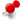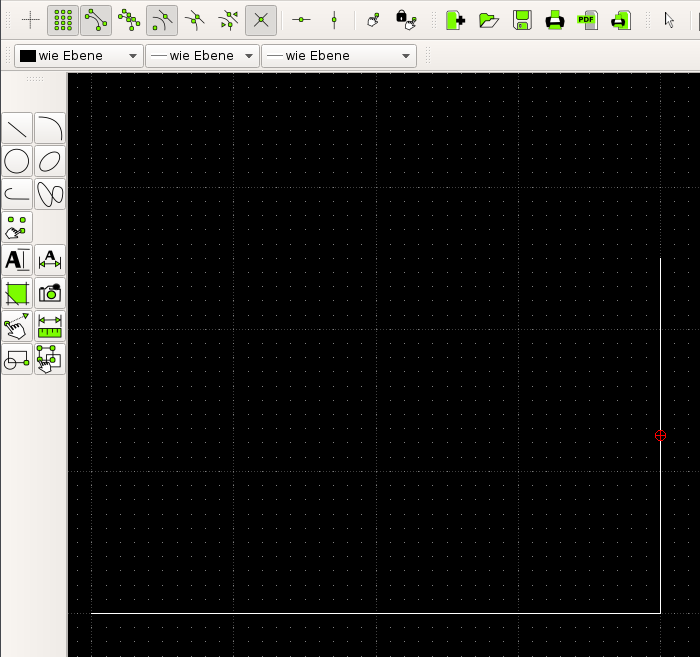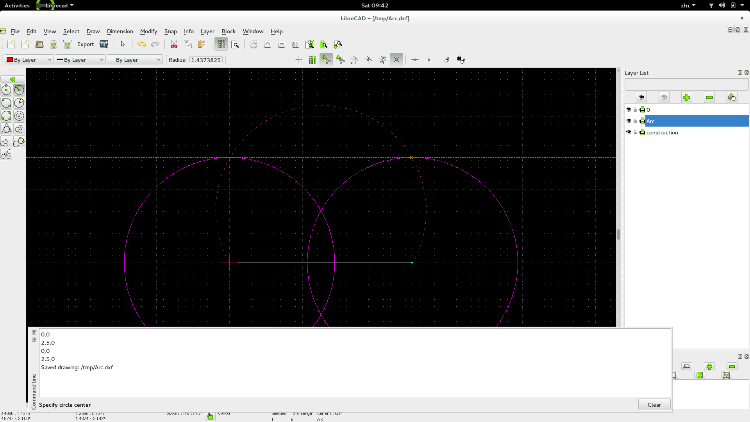# Drawing arc by given length and 2 pointsClassicListThreaded2 messagesI want to draw an arc with length 6 meter and the distance between begin-/endpoint is 2.5 meter. Any suggestions how exactly to do this? Example attached:the vertical line is the distance between begin- and endpoint, the horizontal line is the length of the arc.This post was updated on . I suppose there's no compass-and-straight-edge solution. the arc should cover more than 180 deg, because: 6/(2.5/2) > Pi. Let r be the radius, then, the angle of the arc is 2 * (Pi - ArcSin[2.5/2/r]), and the total arc length is r* (2 * ( Pi - ArcSin[2.5/2/r]) ) = 6 solve this equation to find the radius r is 1.437382... http://www.wolframalpha.com/input/?i=Solve%5B+%28Pi+-+ArcSin%5B1.25%2Fr%5D%29*r*2%3D%3D6%2C+r%5DSee the screenshot. To find the length of an arc (or other primitive entities), just select it, and the selection widget shows the number of entities selected, and the total length of selected. I gotArc.dxf  cupakob wrote I want to draw an arc with length 6 meter and the distance between begin-/endpoint is 2.5 meter. Any suggestions how exactly to do this? Example attached:the vertical line is the distance between begin- and endpoint, the horizontal line is the length of the arc.